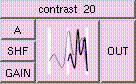CONTRASTTHE BOX'S MAIN HELP: This function can increase or decrease the contrast of A. If SHF is negative and GAIN is > 1 this will increase the contrast. If SHF is positive and GAIN is < 1 the contrast will be decreased. The result will be the same data type as A. A (input): This may be a float, point, or color. The default is float. SHF (input): This must be a float between [-1,1]. This value is added to A. GAIN (input): This must be a float. (A+SHF) is multplied by this input. OUT (output): This function can increase or decrease the contrast of A. The result will be the same data type as A.

<-- BACK TO Math